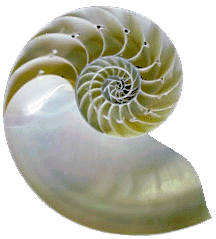Search IntMath
Close

450+ Math Lessons written by Math Professors and Teachers

5 Million+ Students Helped Each Year

1200+ Articles Written by Math Educators and Enthusiasts

Simplifying and Teaching Math for Over 23 Years

# Equiangular Spiral

By M. BourneNautilus Shell
(Image from Tree of Life)

The equation for the equiangular spiral was developed by Rene Descartes (1596-1650) in 1638.

This spiral occurs naturally in many places like sea-shells where the growth of an organism is proportional to the size of the organism.

It's also known as the Logarithmic Spiral due to the way the spiral arms increase in distance from the center at the same ratio.

The general polar equation for the equiangular spiral curve is

r = aeθ cot b

### Example 1: Equiangular Spiral

Let a = 0.5 and b = 1.4 (radians).

Then

r = 0.5 eθ cot 1.4

Here's the graph:

Graph of r = 0.5 eθ cot 1.4, an equiangular spiral.

It is called an "equiangular" spiral because any radius vector makes the same angle with the curve. In this example, b = 1.4 radians, or in degrees, 1.4xx180/pi=80.214^text(o).

We can see that any radius vector makes the angle 80^@ with the curve.

Graph of equiangular spiral showing equal angles cut by radius vectors.

[For background, see the vectors chapter.]

## Spiral of Archimedes

The equiangular spiral is not the same as the "Spiral of Archimedes" which has the form:

r =

This spiral has arms which are equidistant.

### Example 2: Spiral of Archimedes

r = 2θ

Graph of r = 2θ, an Archimedean Spiral.

See this article on finding the length of an Archimedean Sprial, using calculus.

## Problem SolverThis tool combines the power of mathematical computation engine that excels at solving mathematical formulas with the power of GPT large language models to parse and generate natural language. This creates math problem solver thats more accurate than ChatGPT, more flexible than a calculator, and faster answers than a human tutor. Learn More.